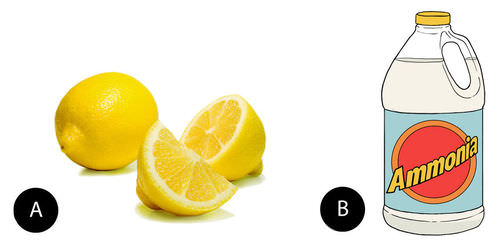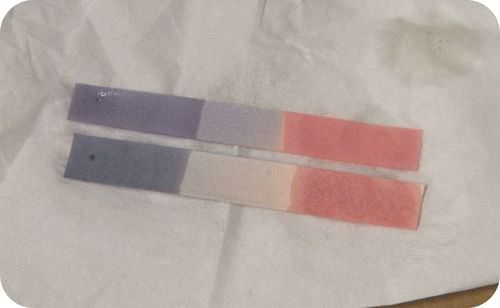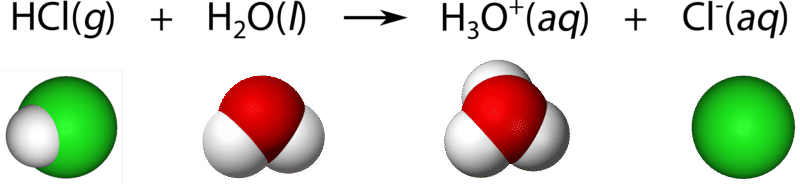# 8.5: Acid-Base Definitions

•• Contributed by Allison Soult
• Senior Lecturer (Chemistry) at University of Kentucky

Learning Outcomes

• Describe the properties of acids and bases.
• Define an acid and a base according to the Arrhenius theory.
• Define an acid and a base according to the Brønsted-Lowry theory.
• Identify the conjugate acid-base pairs in a Brønsted-Lowry acid-base reaction.

## Acid-Base Properties

Acids are very common in some of the foods that we eat. Citrus fruits such as oranges and lemons contain citric acid and ascorbic acid, which is better known as vitamin C (see figure below). Carbonated sodas contain phosphoric acid. Vinegar contains acetic acid. Your own stomach utilizes hydrochloric acid to digest food. Bases are less common as foods, but they are nonetheless present in many household products (see figure below). Many cleaners contain ammonia, a base. Sodium hydroxide is found in drain cleaner. Antacids, which combat excess stomach acid, are comprise of bases such as magnesium hydroxide or sodium hydrogen carbonate.Figure $$\PageIndex{1}$$: (A) Lemons and other citrus fruits contain citric and ascorbic acids. (B) Ammonia is a base that is present in many household cleaners.

### Acids

Acids are a distinct class of compounds because of the properties of their aqueous solutions. Those properties are outlined below.

1. Aqueous solutions of acids are electrolytes, meaning that they conduct an electrical current. Some acids are strong electrolytes because they ionize completely in water. Other acids are weak electrolytes which partially ionize when dissolved in water.
2. Acids have a sour taste. Lemons, vinegar, and sour candies all contain acids.
3. Acids change the color of certain acid-base indicators .Two common indicators are litmus and phenolphthalein. Litmus turns red in the presence of an acid, while phenolphthalein is colorless.
4. Acids react with some metals to yield hydrogen gas.
5. Acids react with bases to produce a salt and water. When equal moles of an acid and a base are combined, the acid is neutralized by the base. Water and an ionic compound called a salt are produced.

### Bases

Bases have properties that mostly contrast with those of acids.

1. Aqueous solutions of bases are also electrolytes. Bases can be either strong or weak, just as acids can.
2. Bases often have a bitter taste and are found in foods less frequently than acids. Many bases, like soaps, are slippery to the touch.
3. Bases also change the color of indicators. Litmus turns blue in the presence of a base (see figure below), while phenolphthalein turns pink.
4. Bases do not react with metals in the way that acids do.
5. Bases react with acids to produce a salt and water.Figure $$\PageIndex{2}$$: Litmus paper has be treated with the plant dye called litmus. It turns red in the presence of an acid and blue in the presence of a base.

## Arrhenius Acids and Bases

The Swedish chemist Svante Arrhenius (1859 - 1927) was the first to propose a theory to explain the observed behavior of acids and bases. Because of their ability to conduct a current, he knew that both acids and bases contained ions in solution. An Arrhenius acid is a compound which ionizes to yield hydrogen ions $$\left( \ce{H^+} \right)$$ in aqueous solution. An Arrhenius base is a compound which ionizes to yield hydroxide ions $$\left( \ce{OH^-} \right)$$ in aqueous solution.

### Arrhenius Acids

Acids are molecular compounds with ionizable hydrogen atoms. Only hydrogen atoms that are part of a highly polar covalent bond are ionizable. The hydrogen atom is attracted to the lone pair of electrons in a water molecule when $$\ce{HCl}$$ is dissolved in water. The result is that the $$\ce{H-Cl}$$ bond breaks, with both bonding electrons remaining with the $$\ce{Cl}$$, forming a chloride ion. The $$\ce{H^+}$$ ion attaches to the water molecule, forming a polyatomic ion called the hydronium ion. The hydronium ion $$\left( \ce{H_3O^+} \right)$$ can be thought of as a water molecule with an attached hydrogen ion.Equations showing the ionization of an acid in water are frequently simplified by omitting the water molecule.

$\ce{HCl} \left( g \right) \rightarrow \ce{H^+} \left( aq \right) + \ce{Cl^-} \left( aq \right)$

This is merely a simplification of the previous equation, but it is commonly used. Any hydrogen ions in an aqueous solution will be attached to water molecules as hydronium ions, $$\ce{H_3O^+}$$, even if it is written as $$\ce{H^+}$$.

Not all hydrogen atoms in molecular compounds are ionizable. In methane $$\left( \ce{CH_4} \right)$$, the hydrogen atoms are covalently bonded to carbon in bonds that are only slightly polar. The hydrogen atoms are not capable of ionizing, and methane has no acidic properties. Acetic acid $$\left( \ce{CH_3COOH} \right)$$ (see figure below) belongs to a class of acids called carboxylic acids. There are four hydrogen atoms in the molecule, but only the one hydrogen that is bonded to an oxygen atom is ionizable.Figure $$\PageIndex{3}$$: The $$\ce{O-H}$$ bond can be ionized to yield the $$\ce{H^+}$$ ion and the acetate ion. The other hydrogen atoms in this molecule are not acidic.

The table below lists some of the more common acids.

Table $$\PageIndex{1}$$: Common Acids
Acid Name Formula
hydrochloric acid $$\ce{HCl}$$
nitric acid $$\ce{HNO_3}$$
sulfuric acid $$\ce{H_2SO_4}$$
phosphoric acid $$\ce{H_3PO_4}$$
acetic acid $$\ce{CH_3COOH}$$
hypochlorous acid $$\ce{HClO}$$

A monoprotic acid is an acid that contains only one ionizable hydrogen. Hydrochloric acid and acetic acid are monoprotic acids. A polyprotic acid is an acid that contains multiple ionizable hydrogens. Most common polyprotic acids are either diprotic (such as $$\ce{H_2SO_4}$$), or triprotic (such as $$\ce{H_3PO_4}$$).

### Arrhenius Bases

Bases are ionic compounds which yield the hydroxide ion $$\left( \ce{OH^-} \right)$$ upon dissociating in water. The table below lists several of the more common bases.

Table $$\PageIndex{2}$$: Common Bases
Base Name Formula
Sodium hydroxide $$\ce{NaOH}$$
Potassium hydroxide $$\ce{KOH}$$
Magnesium hydroxide $$\ce{Mg(OH)_2}$$
Calcium hydroxide $$\ce{Ca(OH)_2}$$

All of the bases listed in the table are solids at room temperature. Upon dissolving in water, each dissociates into a metal cation and the hydroxide ion.

$\ce{NaOH} \left( s \right) \overset{\ce{H_2O}}{\rightarrow} \ce{Na^+} \left( aq \right) + \ce{OH^-} \left( aq \right)$

Sodium hydroxide is a very caustic substance also known as lye. Lye is used as a rigorous cleaner and is an ingredient in the manufacture of soaps. Care must be taken with strong bases like sodium hydroxide, as exposure can lead to severe burns (see figure below).Figure $$\PageIndex{4}$$: This foot has sever burns due to prolonged contact with a solution of sodium hydroxide, also known as lye.

## Brønsted-Lowry Acids and Bases

The Arrhenius definition of acids and bases is somewhat limited. There are some compounds whose properties suggest that they are either acidic or basic, but which do not qualify according to the Arrhenius definition. An example is ammonia $$\left( \ce{NH_3} \right)$$. An aqueous solution of ammonia turns litmus blue, reacts with acids, and displays various other properties that are common for bases. However, it does not contain the hydroxide ion. In 1923, a broader definition of acids and bases was independently proposed by the Danish chemist Johannes Brønsted (1879 - 1947) and the English chemist Thomas Lowry (1874 - 1936). A Brønsted-Lowry acid is a molecule or ion that donates a hydrogen ion in a reaction. A Brønsted-Lowry base is a molecule or ion that accepts a hydrogen ion in a reaction. Because the most common isotope of hydrogen consists of a single proton and a single electron, a hydrogen ion (in which the single electron has been removed) is commonly referred to as a proton. As a result, acids and bases are often called proton donors and proton acceptors, respectively, according to the Brønsted-Lowry definition. All substances that are categorized as acids and bases under the Arrhenius definition are also define as such under the Brønsted-Lowry definition. The new definition, however, includes some substances that are left out according to the Arrhenius definition.

### Brønsted-Lowry Acid-Base Reactions

An acid-base reaction according the Brønsted-Lowry definition is a transfer of a proton from one molecule or ion to another. When ammonia is dissolved in water, it undergoes the following reversible reaction.

$\begin{array}{ccccccc} \ce{NH_3} \left( aq \right) & + & \ce{H_2O} \left( l \right) & \rightleftharpoons & \ce{NH_4^+} \left( aq \right) & + & \ce{OH^-} \left( aq \right) \\ \text{base} & & \text{acid} & & \text{acid} & & \text{base} \end{array}$

In this reaction, the water molecule is donating a proton to the ammonia molecule. The resulting products are the ammonium ion and the hydroxide ion. The water is acting as a Brønsted-Lowry acid, while the ammonia is acting as a Brønsted-Lowry base. The hydroxide ion that is produced causes the solution to be basic.

We can also consider the reverse reaction in the above equation. In that reaction, the ammonium ion donates a proton to the hydroxide ion. The ammonium ion is a Brønsted-Lowry acid, while the hydroxide ion is a Brønsted-Lowry base. Most Brønsted-Lowry acid-base reactions can be analyzed in this way. There is one acid and one base as reactants, and one acid and one base as products.

In the above reaction, water acted as an acid, which may seem a bit unexpected. Water can also act as a base in a Brønsted-Lowry acid-base reaction, as long as it reacts with a substance that is a better proton donor. Shown below is the reaction of water with the hydrogen sulfate ion.

$\begin{array}{ccccccc} \ce{HSO_4^-} \left( aq \right) & + & \ce{H_2O} \left( l \right) & \rightleftharpoons & \ce{H_3O^+} \left( aq \right) & + & \ce{SO_4^{2-}} \left( aq \right) \\ \text{acid} & & \text{base} & & \text{acid} & & \text{base} \end{array}$

Water is capable of being either an acid or a base, a characteristic called amphoterism. An amphoteric substance is one that is capable of acting as either an acid or a base by donating or accepting hydrogen ions.

### Conjugate Acids and Bases

When a substance that is acting as a Brønsted-Lowry acid donates its proton, it becomes a base in the reverse reaction. In the reaction above, the hydrogen sulfate ion $$\left( \ce{HSO_4^-} \right)$$ donates a proton to water and becomes a sulfate ion $$\left( \ce{SO_4^{2-}} \right)$$. The $$\ce{HSO_4^-}$$ and the $$\ce{SO_4^{2-}}$$ are linked to one another by the presence or absence of the $$\ce{H^+}$$ ion. A conjugate acid-base pair is a pair of substances related by the loss or gain of a single hydrogen ion. A conjugate acid is the particle produced when a base accepts a proton. The hydrogen sulfate ion is the conjugate acid of the sulfate ion. A conjugate base is the particle produced when an acid donates a proton. The sulfate ion is the conjugate base of the hydrogen sulfate ion.

A typical Brønsted-Lowry acid-base reaction contains two conjugate acid-base pairs as shown below.

$\ce{HNO_2} \left( aq \right) \ce{PO_4^{3-}} \left( aq \right) \rightleftharpoons \ce{NO_2^-} \left( aq \right) + \ce{HPO_4^{2-}} \left( aq \right)$

One conjugate acid-base pair is $$\ce{HNO_2}/\ce{NO_2^-}$$, while the other pair is $$\ce{HPO_4^{2-}}/\ce{PO_4^{3-}}$$.

The buffer systems in blood have conjugate acid-base pairs which help maintain the correct acid and base concentration in blood.

Table $$\PageIndex{3}$$: Acid-Base Definitions
Type Acid Base
Arrhenius $$\ce{H^+}$$ ions in solution $$\ce{OH^-}$$ ions in solution
Brønsted-Lowry $$\ce{H^+}$$ donor $$\ce{H^+}$$ acceptor